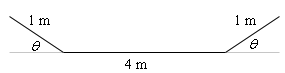Paul's Online Notes
Home / Calculus I / Applications of Derivatives / More Optimization Problems
Show Mobile Notice Show All Notes Hide All Notes
Mobile Notice
You appear to be on a device with a "narrow" screen width (i.e. you are probably on a mobile phone). Due to the nature of the mathematics on this site it is best views in landscape mode. If your device is not in landscape mode many of the equations will run off the side of your device (should be able to scroll to see them) and some of the menu items will be cut off due to the narrow screen width.
Assignment Problems Notice
Please do not email me to get solutions and/or answers to these problems. I will not give them out under any circumstances nor will I respond to any requests to do so. The intent of these problems is for instructors to use them for assignments and having solutions/answers easily available defeats that purpose.

If you are looking for some problems with solutions you can find some by clicking on the "Practice Problems" link above.

### Section 4.9 : More Optimization

1. We want to construct a window whose bottom is a rectangle and the top of the window is an equilateral triangle. If we have 75 inches of framing material what are the dimensions of the window that will let in the most light?
2. We want to construct a window whose middle is a rectangle and the top and bottom of the window are equilateral triangles. If we have 4 feet of framing material what are the dimensions of the window that will let in the most light?
3. We want to construct a window whose middle is a rectangle, the top of the window is a semicircle and the bottom of the window is an equilateral triangle. If we have 1500 cm of framing material what are the dimensions of the window that will let in the most light?
4. Determine the area of the largest rectangle that can be inscribed in a circle of radius 5.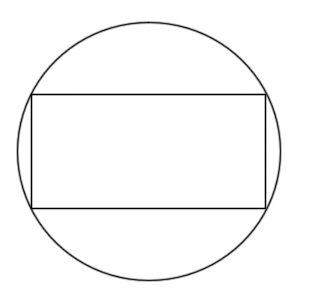5. Determine the area of the largest rectangle whose base is on the $$x$$-axis and the top two corners lie on semicircle of radius 16.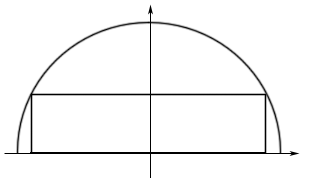6. Determine the area of the largest rectangle whose base is on the $$x$$-axis and the top two corners lie $$y = 4 - {x^2}$$.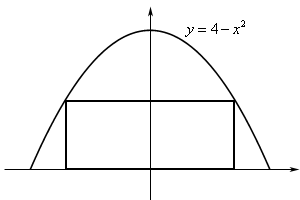7. Find the point(s) on $$\displaystyle \frac{{{x^2}}}{4} + \frac{{{y^2}}}{{36}} = 1$$ that are closest to $$\left( {0,1} \right)$$.
8. Find the point(s) on $$x = {y^2} - 8$$ that are closest to $$\left( {5,0} \right)$$.
9. Find the point(s) on $$y = 2 - {x^2}$$ that are closest to $$\left( {0, - 3} \right)$$.
10. A 6 ft piece of wire is cut into two pieces. One piece is bent into an equilateral triangle and the other will be bent into a rectangle with one side twice the length of the other side. Determine where, if anywhere, the wire should be cut to minimize the area enclosed by the two figures.
11. A 250 cm piece of wire is cut into two pieces. One piece is bent into an equilateral triangle and the other will be bent into circle. Determine where, if anywhere, the wire should be cut to maximize the area enclosed by the two figures.
12. A 250 cm piece of wire is cut into two pieces. One piece is bent into an equilateral triangle and the other will be bent into circle. Determine where, if anywhere, the wire should be cut to minimize the area enclosed by the two figures.
13. A 4 m piece of wire is cut into two pieces. One piece is bent into a circle and the other will be bent into a rectangle with one side three times the length of the other side. Determine where, if anywhere, the wire should be cut to maximize the area enclosed by the two figures.
14. A line through the point $$\left( { - 4,1} \right)$$ forms a right triangle with the $$x$$-axis and $$y$$-axis in the 2nd quadrant. Determine the equation of the line that will minimize the area of this triangle.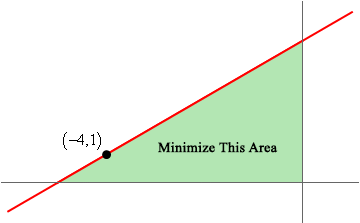15. A line through the point $$\left( {3,3} \right)$$ forms a right triangle with the $$x$$-axis and $$y$$-axis in the 1st quadrant. Determine the equation of the line that will minimize the area of this triangle.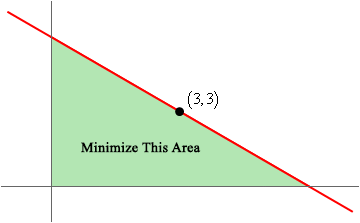16. A piece of pipe is being carried down a hallway that is 14 feet wide. At the end of the hallway there is a right-angled turn and the hallway narrows down to 6 feet wide. What is the longest pipe (always keeping it horizontal) that can be carried around the turn in the hallway?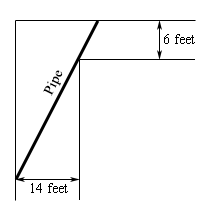17. A piece of pipe is being carried down a hallway that is 9 feet wide. At the end of the hallway there is a right-angled turn and the hallway widens up to 21 feet wide. What is the longest pipe (always keeping it horizontal) that can be carried around the turn in the hallway?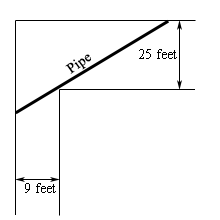18. Two poles, one 15 meters tall and one 10 meters tall, are 40 meters apart. A length of wire is attached to the top of each pole and it is staked to the ground somewhere between the two poles. Where should the wire be staked so that the minimum amount of wire is used?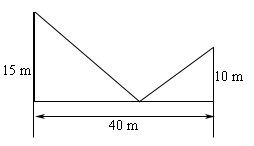19. Two poles, one 2 feet tall and one 5 feet tall, are 3 feet apart. A length of wire is attached to the top of each pole and it is staked to the ground somewhere between the two poles. Where should the wire be staked so that the minimum amount of wire is used?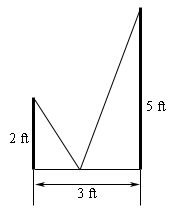20. Two poles, one 15 meters tall and one 10 meters tall, are 40 meters apart. A length of wire is attached to the top of each pole and it is staked to the ground somewhere between the two poles. Where should the wire be staked so that the angle formed by the two pieces of wire at the stake is a maximum?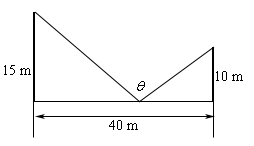21. Two poles, one 34 inches tall and one 17 inches tall, are 3 feet apart. A length of wire is attached to the top of each pole and it is staked to the ground somewhere between the two poles. Where should the wire be staked so that the angle formed by the two pieces of wire at the stake is a maximum?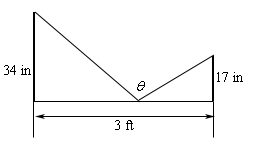22. A trough for holding water is to be formed as shown in the figure below. Determine the angle $$\theta$$ that will maximize the amount of water that the trough can hold.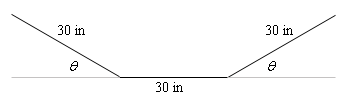23. A trough for holding water is to be formed as shown in the figure below. Determine the angle $$\theta$$ that will maximize the amount of water that the trough can hold.